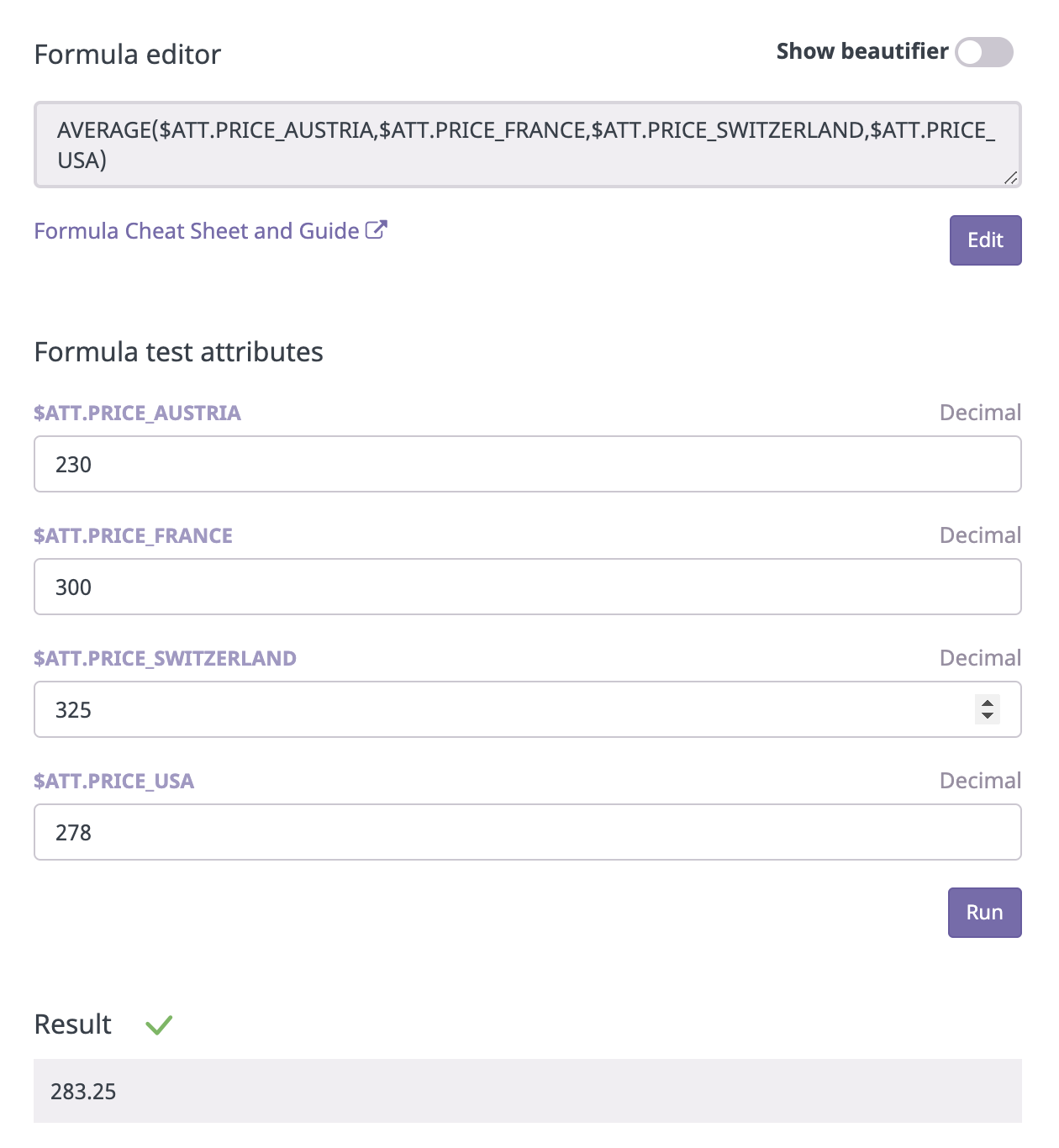# AVERAGE Operation

## How to use the AVERAGE operation for Computed Attributes

### Definition

The AVERAGE operation finds the average in a numerical dataset.

AVERAGE(21,35,7)

Result:
21

### Syntax Guide

AVERAGE(value1, [value2], ...)

value1 - The first numerical value or range to consider when calculating the average value

value2, ... - [ OPTIONAL ] - Additional numerical values or ranges to consider when calculating the average value### Compatible Attribute Types

• Integer attributes
• Decimal attributes